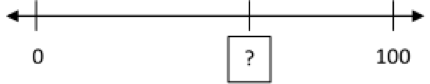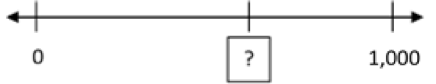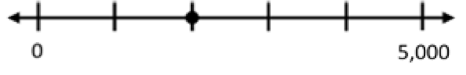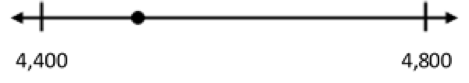# Place Value, Rounding, Addition, and Subtraction

## Objective

Locate multi-digit numbers on a number line and explain their placement.

## Common Core Standards

### Core Standards

?

• 4.NBT.A.2 — Read and write multi-digit whole numbers using base-ten numerals, number names, and expanded form. Compare two multi-digit numbers based on meanings of the digits in each place, using >, =, and < symbols to record the results of comparisons.

• 4.NBT.A.3 — Use place value understanding to round multi-digit whole numbers to any place.

?

• 2.MD.B.6

## Criteria for Success

?

1. Understand that number lines have specific conventions: the use of a single position to represent a whole number and the use of marks to indicate those positions.
2. Understand that a number line is like a ruler in that consecutive whole numbers are 1 unit apart (where that unit can be 1, 10, 25, etc.), thus they need to consider the distances between positions and segments when identifying missing numbers.
3. Identify and represent whole numbers on a number line diagram including:
• Those with and without intervals (i.e., “open” number lines)
• Those with intervals marked but not labeled
• Those not starting at 0
• Those with intervals greater than 1

#### Fishtank Plus

Subscribe to Fishtank Plus to unlock access to additional resources for this lesson, including:

• Problem Set
• Student Handout Editor
• Google Classrom Integration
• Vocabulary Package

## Anchor Tasks

?

### Problem 1

1. Look at the line below:Based on where 0 and 100 are, what number do you think the question mark is on? Explain your choice.

1. Look at the line below:Based on where 0 and 1,000 are, what number do you think the question mark is on?

#### Guiding Questions

Create a free account or sign in to access the Guiding Questions for this Anchor Problem.

#### References

John A. Van de Walle Teaching Student-Centered Mathematics: Developmentally Appropriate Instruction for Grades 3-5 (Volume II)Activity 10.2

Van de Walle, John A. Teaching Student-Centered Mathematics: Developmentally Appropriate Instruction for Grades 3-5 (Volume II). Pearson, 2nd edition, 2013.

Modified by Fishtank Learning, Inc.

### Problem 2

1. What is the location of the following point?1. What is the location of the following point?#### Guiding Questions

Create a free account or sign in to access the Guiding Questions for this Anchor Problem.

### Problem 3

1. Plot 27,361 on a number line from 27,360 to 27,370.
2. Plot 27,361on a number line from 27,300 to 27,400.
3. Plot 27,361on a number line from 27,000 to 28,000.
4. Plot 27,361on a number line from 20,000 to 30,000.

#### Guiding Questions

Create a free account or sign in to access the Guiding Questions for this Anchor Problem.

## Problem Set & Homework

• Problem Set Answer Key
• Homework Answer Key

### Discussion of Problem Set

• What’s different about the values in #2(a) and (b)? How is that difference represented on the number line?
• Look #3. How did you decide what to make your number lines look like to place 156,780 on them? How do your number lines look similar and different (to each other and to other students’ number lines)?
• Look at #6. How did you determine where each value would go?
• What values fit both criteria in #9? How did you figure that out?

## Target Task

?

Complete each of the following.

a.   Label all the other tick marks between 8,260 and 8,270. Then plot 8,263.

b.   Plot 8,200 and 8,300 on the two outermost spots on the number line below. Label the rest of the tick marks. Then plot 8,263.

c.   Plot 8,000 and 9,000 on the two outermost spots on the number line below. Label the rest of the tick marks. Then plot 8,263.

#### Mastery Response

Create a free account or sign in to view Mastery Response

## Additional Practice#### Unit Practice

With Fishtank Plus you can access our Daily Word Problem Practice and our content-aligned Fluency Activities created to help students strengthen their application and fluency skills.

View Preview